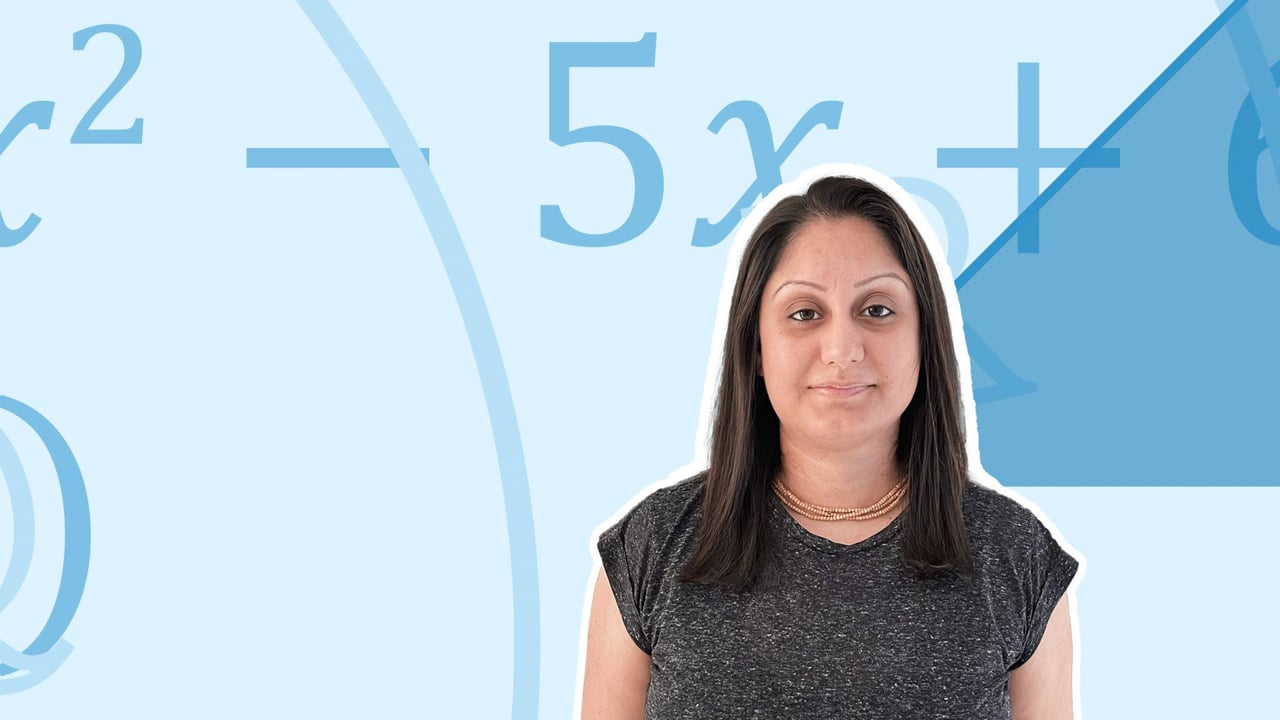Chapter overviewMaths

Exam board

AQA

Number

Algebra

Graphs

Ratio proportion and rates of change

Shapes and area

Angles and geometry

Trigonometry

Probability

Statistics

Maths

# Writing formulae and equations from word problems0%

Summary

# Writing formulae and equations from word problems

## In a nutshell

You should be able to form expressions or formulae from information given in a question. Start by giving the unknown quantities a letter name, e.g. $x$. Then use the information in the question to create your expression or formula.

Note: Remember to substitute into the formula to check that is works.

## Make an expression

To make an expression, read the information given, give the unknown quantity a letter name and use your knowledge of algebra to write the correct expression.

##### Example 1

Fred is $x$ years old. George is $4$ years older than Fred. Harry is twice as old as George. Write expressions, in terms of $x$ for their ages.

 Fred ​$x$​​ George ​$x+4$​​ Harry ​$2(x+4) = 2x+8$​​

## Make a formula

To make a formula, read the information given, give the unknown quantity a letter name and use your knowledge of algebra to give the formula.

##### Example 2

The total cost, $C$, of a mobile phone bill is the cost of text messages, $t$, and the cost of calling, $m$. Texts are $5p$ each, and each minute of a call is $10p$. Write a formula for the cost, $C$, of the mobile phone bill.

$\underline{C= 0.05t+0.1m}$

## Use a formula to calculate the unknown quantity

Create a formula, as above, then substitute in for the variables in the formula to calculate the result.

##### Example 3

A restaurant charges $£C$ for delivering pizzas, through a delivery app. They charge a standard fee of $£1.50$ and an additional $80p$ for each mile, m, travelled. Write a formula for $C$ and work out the charge for a $5$ mile delivery.

$C=0.8m+1.5$

Note: Remember to convert to the same units if necessary, in this case $80p$ has been converted to $£0.8$.

Now use the formula by substituting $m=5$ to calculate the cost.​

$C = 0.8 \times 5 + 1.5 = 5.5$

The total cost is $\underline{£5.50}$

## Want to find out more? Check out these other lessons!

Writing and using formulae

FAQs

• Question: How can you check if a formula you created works?

Answer: After creating a formula, you can check it works by substituting values to make sure that the answer is as expected.

• Question: How can I create a formula in algebra?

Answer: A formula can be created by analysing a questions, given in context. Letters can be used for the unknown variables to help create the formula.

Theory

Exercises